Next: DOMAIN CIRCUMSCRIPTION Up: CIRCUMSCRIPTION-A FORM OF NONMONOTONIC Previous: MISSIONARIES AND CANNIBALS

# THE FORMALISM OF CIRCUMSCRIPTION

Let A be a sentence of first order logic containing a predicate symbol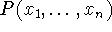which we will write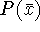. We write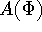for the result of replacing all occurrences of P in A by the predicate expression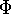. (As well as predicate symbols, suitable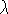-expressions are allowed as predicate expressions).

Definition. The circumscription of P in A(P) is the sentence schema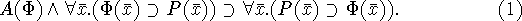(1) can be regarded as asserting that the only tuples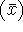that satisfy P are those that have to -- assuming the sentence A. Namely, (1) contains a predicate parameterfor which we may subsitute an arbitrary predicate expression. (If we were using second order logic, there would be a quantifier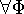in front of (1).) Since (1) is an implication, we can assume both conjuncts on the left, and (1) lets us conclude the sentence on the right. The first conjunctexpresses the assumption thatsatisfies the conditions satisfied by P, and the second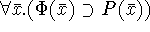expresses the assumption that the entities satisfyingare a subset of those that satisfy P. The conclusion asserts the converse of the second conjunct which tells us that in this case,and P must coincide.

We write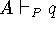if the sentence q can be obtained by deduction from the result of circumscribing P in A. As we shall see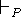is a nonmonotonic form of inference, which we shall call circumscriptive inference.

A slight generalization allows circumscribing several predicates jointly; thus jointly circumscribing P and Q in A(P,Q) leads to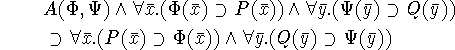in which we can simultaneously substitute forand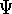. The relation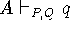is defined in a corresponding way. Although we don't give examples of joint circumscription in this paper, we believe it will be important in some AI applications.

Consider the following examples:

Example 1. In the blocks world, the sentence A may be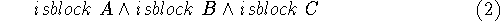asserting that A, B and C are blocks. Circumscribing isblock in (2) gives the schema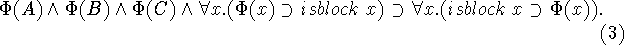If we now substitute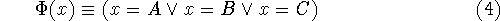into (3) and use (2), the left side of the implication is seen to be true, and this gives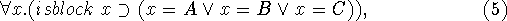which asserts that the only blocks are A, B and C, i.e. just those objects that (2) requires to be blocks. This example is rather trivial, because (2) provides no way of generating new blocks from old ones. However, it shows that circumscriptive inference is nonmonotonic since if we adjoin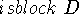to (2), we will no longer be able to infer (5).

Example 2. Circumscribing the disjunction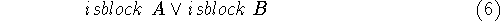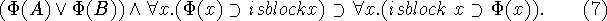We may then substitute successively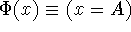and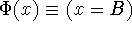, and these give respectively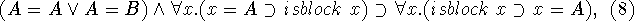which simplifies to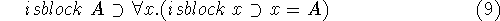and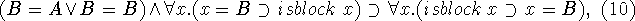which simplifies to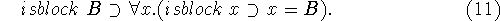(9), (11) and (6) yield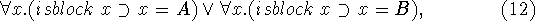which asserts that either A is the only block or B is the only block.

Example 3. Consider the following algebraic axioms for natural numbers, i.e., non-negative integers, appropriate when we aren't supposing that natural numbers are the only objects.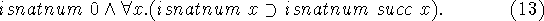Circumscribing isnatnum in (13) yields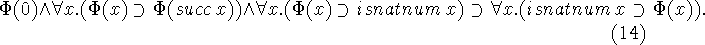(14) asserts that the only natural numbers are those objects that (13) forces to be natural numbers, and this is essentially the usual axiom schema of induction. We can get closer to the usual schema by substituting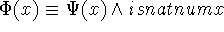. This and (13) make the second conjunct drop out giving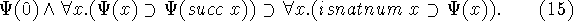Example 4. Returning to the blocks world, suppose we have a predicate on(x,y,s) asserting that block x is on block y in situation s. Suppose we have another predicate above(x,y,s) which asserts that block x is above block y in situation s. We may write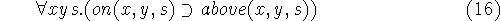and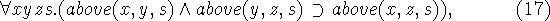i.e. above is a transitive relation. Circumscribing above in (16)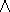(17) gives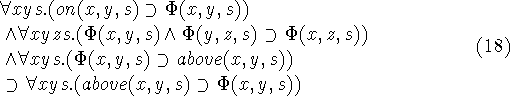which tells us that above is the transitive closure of on.

In the preceding two examples, the schemas produced by circumscription play the role of axiom schemas rather than being just conjectures.Next: DOMAIN CIRCUMSCRIPTION Up: CIRCUMSCRIPTION-A FORM OF NONMONOTONIC Previous: MISSIONARIES AND CANNIBALS

John McCarthy
Tue May 14 00:04:52 PDT 1996